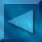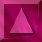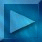Previous : Sampling the densities  Next : The MCMCML estimation algorithm Notations

 The modified Geman & Yang sampler

We use a half-quadratic form of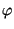: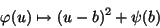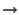auxiliary variable b, auxiliary images Bx, By (gradients w.r.t.  x and y)sampling triplets (X, Bx, By) in an alternated way on X and Bx, Bythe pixels of Bx and By are independent
and the law of X knowing Bx, By is Gaussiansampling X  is made in a single pass (unlike Gibbs and Metropolis samplers),
the processing is independent of the neighbourhood size
which provides a fast algorithm for posterior distribution sampling.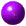Posterior distribution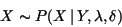Posterior modified Geman & Yang algorithm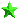Initialization :  We first calculate the Fourier transform F[h4 ] and DCT[Y], and W :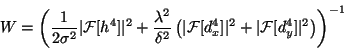The initialization is done using the reconstructed image, obtained with the ICM-DCT algorithm : X0 =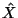.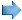Sampling Bx,By with a fixed X The pixels b of Bx and By are independent :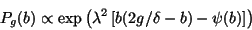Sampling X with fixed Bx,By The distribution of X with known B is Gaussian because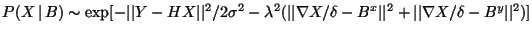diagonalization of the covariance matrix             by a cosine transform  (DCT)            which enables to fulfill symmetric boundary conditions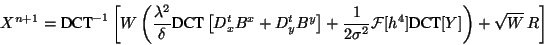where R is an image whose pixels are Gaussian random variables,  with variance 1/2.

Example (64x64 image) :

 X0initialization  X0 =XB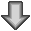Bx 0, By 0BX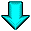iteration 1 X1XBBx 1, By 1BXiteration 2 X2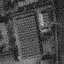etc. Ni iterations for initialization before reaching the equilibrum distributionPrior distribution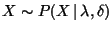Prior modified Geman & Yang algorithmInitialization :  We first calculate the Fourier transform F[h4 ] and W0 :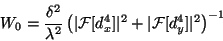The initialization is done using a constant image : X0 = 0.Sampling Bx,By with a fixed X The pixels b of Bx and By are independent :Sampling X with fixed Bx, By  The distribution of X with a known B is Gaussian because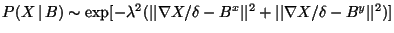diagonalization of the covariance matrix             by a cosine transform  (DCT)            which enables to fulfill symmetric boundary conditions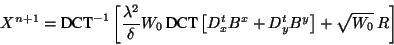where R is an image whose pixels are Gaussian random variables,  with variance 1/2.

Example (64x64 sample) :

 X0initialization  X0 = 0 XBBx 0, By 0BXiteration 1 X1XBBx 1, By 1BXiteration 2 X2etc. Ni iterations for initialization before reaching the equilibrum distribution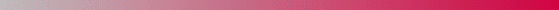André Jalobeanu - 24 Aug 1998